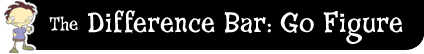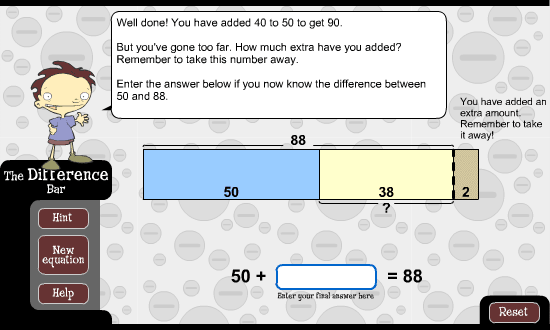﻿ The difference bar: go figure: compensateCompensate

The idea behind this strategy is to add more than is needed in order to find the difference. Then, take away the extra amount added to get the final answer.

For example, let's look at the difference between 50 and 88. This is the same as 50 + ? = 88. First, work out that 50 + 40 is 90. So, add on 40. This is two more than is needed. So, find the final answer by taking 2 away from 40. Therefore, the final answer is 38.